# Symbolic Regression, Genetic Programming… or if Kepler had R

April 2, 2019
By

Want to share your content on R-bloggers? click here if you have a blog, or here if you don't.A few weeks ago we published a post about using the power of the evolutionary method for optimization (see Evolution works!). In this post we will go a step further, so read on…

A problem researchers often face is that they have an amount of data and need to find some functional form, e.g. some kind of physical law, for it.

The standard approach is to try different functional forms, like linear, quadratic or polynomial functions with higher order terms. Also possible is a fourier analysis with trigonometric functions. But all of those approaches are quite limited and it would be nice if we had a program to do this for us and come up with a functional form that is both accurate and parsimonious… well, your prayers were heard!

This approach is called symbolic regression (also sometimes called free-form regression) – a special case of what is called genetic programming – and the idea is to give the algorithm a grammar which defines some basic functional building blocks (like addition, subtraction, multiplication, logarithms, trigonometric functions and so on) and then try different combinations in an evolutionary process which keeps the better terms and recombines them to even more fitting terms. And the end we want to have a nice formula which captures the dynamics of the system without overfitting the noise. The package with which you can do such magic is the `gramEvol` package (on CRAN).

Let us start with a simple example where we first generate some data on the basis of a combination of trig functions:```x <- seq(0, 4*pi, length.out = 201)
y <- sin(x) + cos(x + x)
plot(y)
```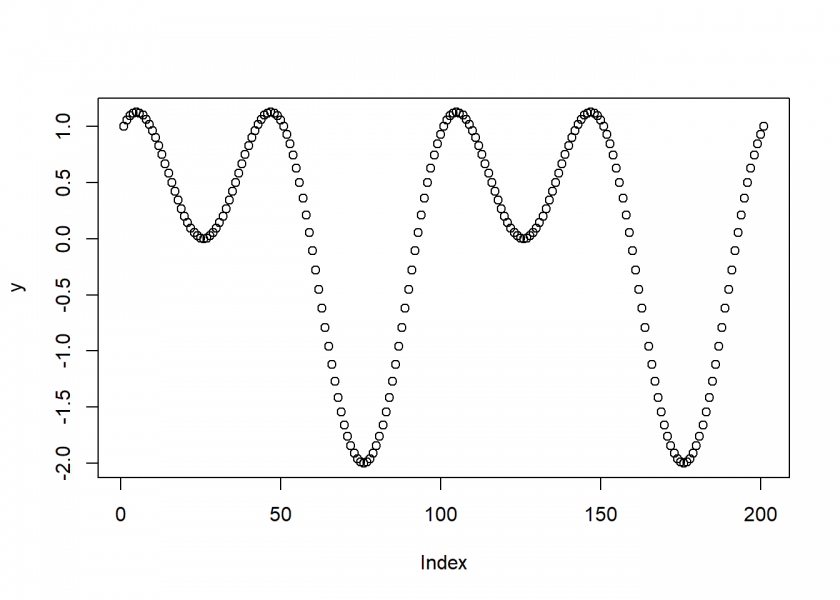We now try to find this functional form by just giving the program the generated data points.

As a first step we load the package and define the grammar, i.e. the allowed functional building blocks (for details how to define your own grammar consult the package’s documentation):

```library("gramEvol")

ruleDef <- list(expr = grule(op(expr, expr), func(expr), var),
func = grule(sin, cos),
op = grule('+', '-', '*'),
var = grule(x))

grammarDef <- CreateGrammar(ruleDef)
grammarDef
##  ::= (, ) | () |
##  ::= `sin` | `cos`
##    ::= "+" | "-" | "*"
##   ::= x
```

Just to give some examples of randomly created formulas from this grammar you could use the `GrammarRandomExpression` function:

```set.seed(123)
GrammarRandomExpression(grammarDef, 6)
## []
## expression(sin(cos(x + x)))
##
## []
## expression(sin(cos(x * x)) + x)
##
## []
## expression((x - cos(x)) * x)
##
## []
## expression(x)
##
## []
## expression(sin(x))
##
## []
## expression(x + x)
```

After that we have to define some cost function which is used to evaluate how good the respective formula is (again, for details please consult the documentation):

```SymRegFitFunc <- function(expr) {
result <- eval(expr)
if (any(is.nan(result)))
return(Inf)
return (mean(log(1 + abs(y - result))))
}
```

The last step is starting the search and see what the algorithm finds:

```set.seed(314)
ge <- GrammaticalEvolution(grammarDef, SymRegFitFunc, terminationCost = 0.1, iterations = 2500, max.depth = 5)
ge
## Grammatical Evolution Search Results:
##   No. Generations:  2149
##   Best Expression:  sin(x) + cos(x + x)
##   Best Cost:        0

plot(y)
points(eval(ge\$best\$expressions), col = "red", type = "l")
```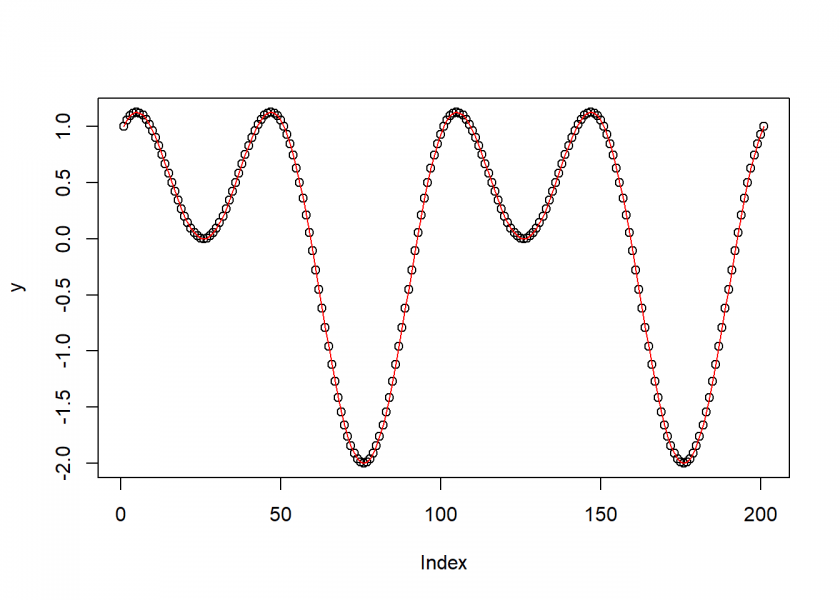Quite impressive, isn’t it? It found the exact same form by just “looking at” the data and trying different combinations of functions, guided by evolution.

Now, in a real world setting you normally don’t have perfect data but you always have some measurement errors (= noise), so we run the example again but this time with some added noise (we use the `jitter` function for that):

```x <- seq(0, 4*pi, length.out = 201)
y <- jitter(sin(x) + cos(x + x), amount = 0.2)
plot(y)
```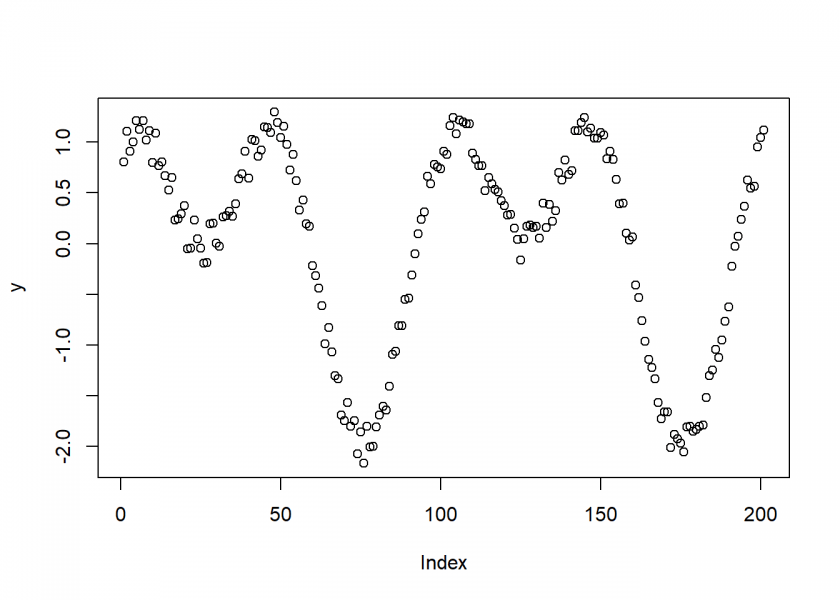And now for the rest of the steps:

```ruleDef <- list(expr = grule(op(expr, expr), func(expr), var),
func = grule(sin, cos),
op = grule('+', '-', '*'),
var = grule(x))

grammarDef <- CreateGrammar(ruleDef)
grammarDef
##  ::= (, ) | () |
##  ::= `sin` | `cos`
##    ::= "+" | "-" | "*"
##   ::= x

SymRegFitFunc <- function(expr) {
result <- eval(expr)
if (any(is.nan(result)))
return(Inf)
return (mean(log(1 + abs(y - result))))
}

set.seed(314)
ge <- GrammaticalEvolution(grammarDef, SymRegFitFunc, terminationCost = 0.1, iterations = 2500, max.depth = 5)
ge
## Grammatical Evolution Search Results:
##   No. Generations:  2149
##   Best Expression:  sin(x) + cos(x + x)
##   Best Cost:        0.0923240003917875

plot(y)
points(eval(ge\$best\$expressions), col = "red", type = "l")
```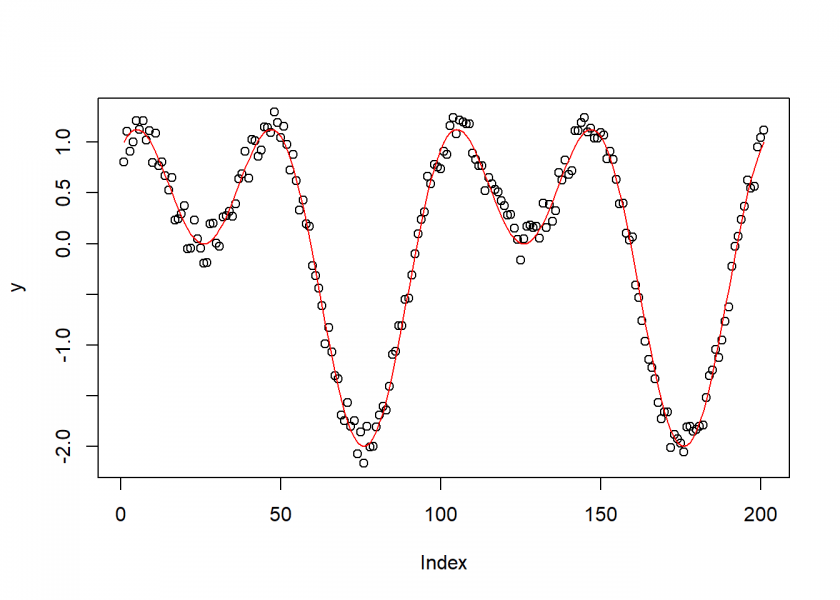Although we added quite some noise the program was still successful in finding the original functional form!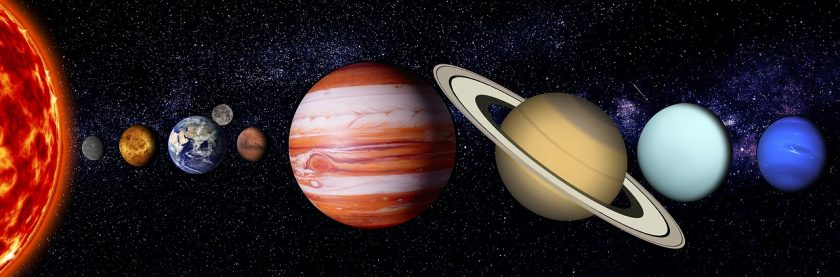Now, we are ready to try something more useful: finding a real physical law of nature! We want to find the relationship between orbital periods and distances from the sun of our solar system.

First we provide the distance and period data, normalised for the earth:

```planets <- c("Venus", "Earth", "Mars", "Jupiter", "Saturn", "Uranus")
distance <- c(0.72, 1.00, 1.52, 5.20, 9.53, 19.10)
period <- c(0.61, 1.00, 1.84, 11.90, 29.40, 83.50)
data.frame(planets, distance, period)
##   planets distance period
## 1   Venus     0.72   0.61
## 2   Earth     1.00   1.00
## 3    Mars     1.52   1.84
## 4 Jupiter     5.20  11.90
## 5  Saturn     9.53  29.40
## 6  Uranus    19.10  83.50
```

And after that we perform just the same steps as above:

```ruleDef <- list(expr = grule(op(expr, expr), func(expr), var),
func = grule(sin, cos, tan, log, sqrt),
op = grule('+', '-', '*', '/', '^'),
var = grule(distance, n),
n = grule(1, 2, 3, 4, 5, 6, 7, 8, 9))

grammarDef <- CreateGrammar(ruleDef)
grammarDef
##  ::= (, ) | () |
##  ::= `sin` | `cos` | `tan` | `log` | `sqrt`
##    ::= "+" | "-" | "*" | "/" | "^"
##   ::= distance |
##     ::= 1 | 2 | 3 | 4 | 5 | 6 | 7 | 8 | 9

SymRegFitFunc <- function(expr) {
result <- eval(expr)
if (any(is.nan(result)))
return(Inf)
return (mean(log(1 + abs(period - result))))
}

set.seed(2)
suppressWarnings(ge <- GrammaticalEvolution(grammarDef, SymRegFitFunc, terminationCost = 0.05))
ge
## Grammatical Evolution Search Results:
##   No. Generations:  42
##   Best Expression:  sqrt(distance) * distance
##   Best Cost:        0.0201895728693589
```

Wow, the algorithm just rediscovered the third law of Kepler in no time!

The square of the orbital period of a planet is directly proportional to the cube of the semi-major axis of its orbit.

If only Kepler could have used R!R-bloggers.com offers daily e-mail updates about R news and tutorials about learning R and many other topics. Click here if you're looking to post or find an R/data-science job.
Want to share your content on R-bloggers? click here if you have a blog, or here if you don't.